### Base rates for US cyclical companies

Case Notes 32: This article compiles the base rates for the key metrics used in deriving the WACC for the US steel, home building, and building products sectors.

I invest in cyclical companies and this requires me to value such companies. I use the FCFF model for this with the WACC as one of the key inputs.

In valuing cyclical companies, I determined the present value of the free cash flow over the cycle. For consistency, the discount rate used should then be the rate over the cycle.

This is where the base rates come in. The duration of the cycle varies from sector to sector. As such it was more appropriate that I compiled the data from 2000 to 2022. This would provide flexibility in catering to different cycle durations.

Join me as I share my valuation model and demonstrate how to use the base rates to derive the cyclical discount rates. I will illustrate them with examples from the US home building, steel and building materials sectors.

Should you go and invest in them? Well, read my Disclaimer.

## Contents

• How to value cyclical companies
• Cost of Capital
• WACC base rates
• Debt
• Impact of using cyclical values
• Conclusion
 This blog is reader-supported. When you buy through links in the post, the blog will earn a small commission. The payment comes from the retailer and not from you. Learn more.

## How to value cyclical companies

“Cyclical and commodity companies share a common feature, insofar as their value is often more dependent on the movement of a macro variable (the commodity price or the growth in the underlying economy) than it is on firm-specific characteristics…the biggest problem we face in valuing companies tied to either is that the earnings and cash flows reported in the most recent year are a function of where we are in the cycle, and extrapolating those numbers into the future can result in serious misvaluation.” Damodaran

To overcome the cyclical issue, we have to normalize the performance over the cycle. Damodaran suggested 3 ways to do this:
• Take the average values over the cycle.
• Take the current revenue and determine the earnings by multiplying it with the normalized margins.
• Use industry-normalized values if there is insufficient company data.

### My approach

I follow these steps when valuing cyclical companies:
• Verify that the company is cyclical.
• Identify the latest cycle.
• Estimate the normalized values over the latest cycle.
• Value the operating assets using the normalized FFCF and discount rate.

To verify that the company is cyclical, some investors look at how stock prices move. However, I believe that looking at whether the business fundamentals - revenue or earnings - are cyclical is a better approach.

I start by estimating the correlation between the revenue and the appropriate macroeconomic factor. This factor could be the commodity price or some sector factor such as the Housing Starts. I look for a correlation of at least 70 % as this meant that the macroeconomic factor could explain half of the revenue changes.

Chart 1 to 3 illustrates some examples of the cycles.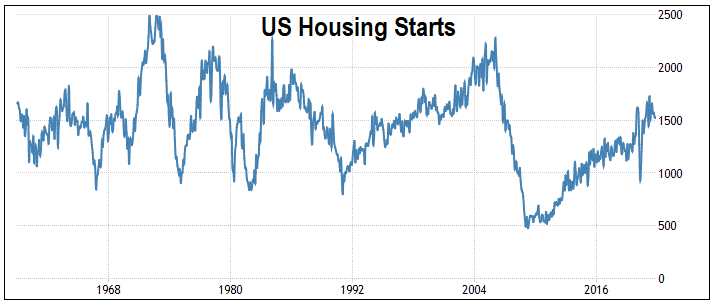Chart 1: US Housing Starts.    Source: Trading Economics.com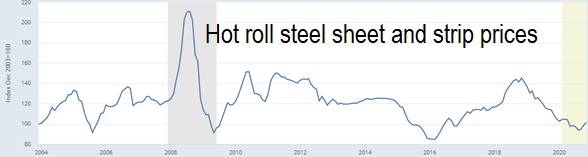Chart 2: US Hot Roll Steel Prices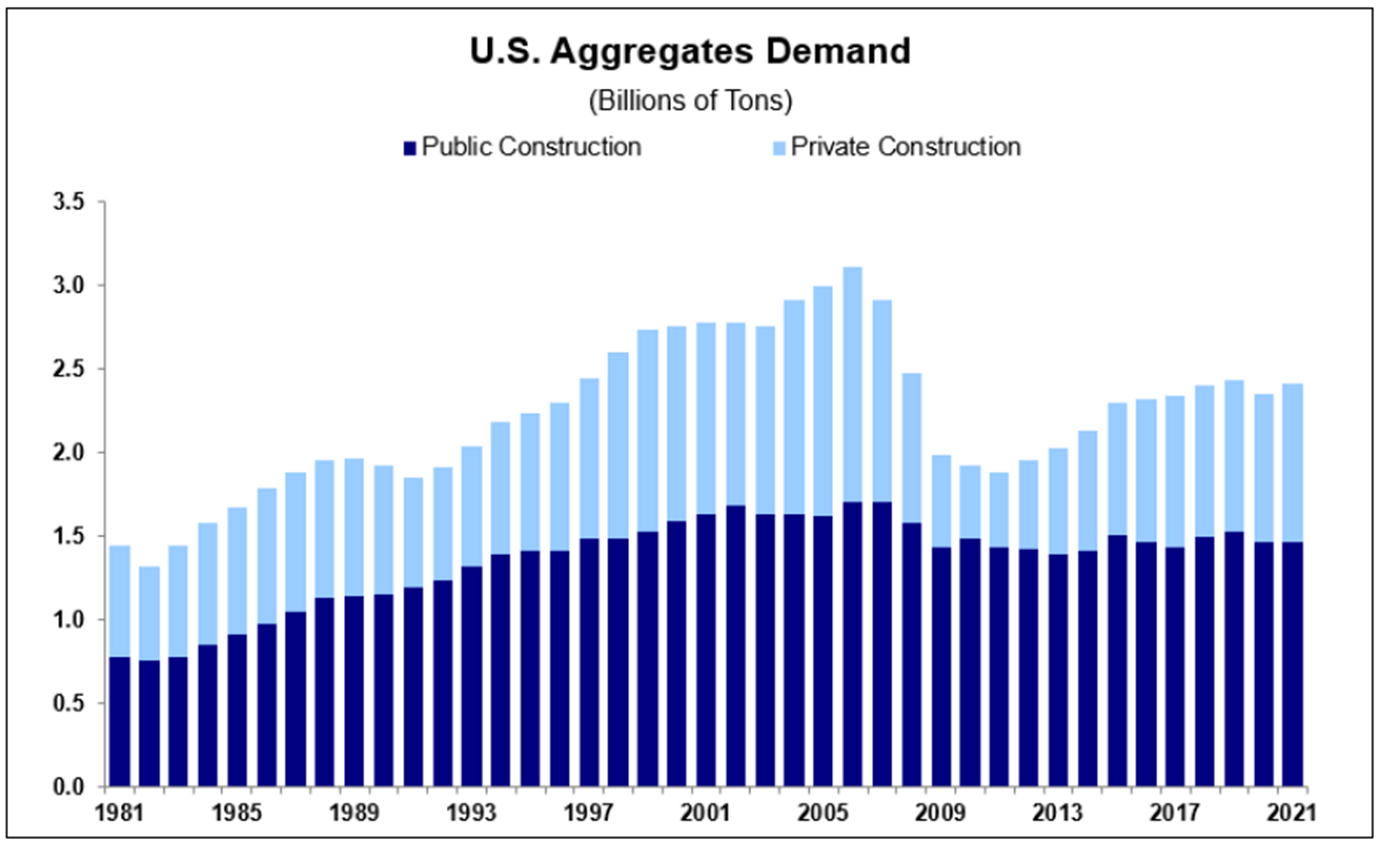Chart 3: US Aggregates Demand

I next select the cycle period so that I can determine the normalized values. The best is that there are data to compute one peak-to-peak average.

The goal is to determine the normalized values for all the parameters required for valuation. This covers the cash flows, the growth rate, and the discount rate.

### My valuation model

I used the single-stage FCFF model to determine the intrinsic value:

Value of operating assets = FCFF(1 + g)/(WACC - g) .

Value of the firm = value of operating assets + value of non-operating assets.

Where:

FCFF = free cashflow to the Firm = EBIT(1-t)(1-Re).

EBIT = earnings before interest and taxes = Revenue x (Gross profit margin - SGA margin).

t = tax rate.

g = growth rate = Return × Reinvestment rate.

Return = EBIT(1-t)/TCE.

TCE = total capital employed = total Equity + Debt - Non-operating assets.

Reinvestment = CAPEX - Depreciation & Amortization + increase in Net Working Capital.

Re = Reinvestment rate = Reinvestment/EBIT(1-t).

WACC = weighted average cost of capital.

You will appreciate that the relevant values for all the parameters should be the normalized values over the cycle.

I used several ways to estimate the normalized values. Refer to the various Seeking Alpha articles on the  steel, home building, and home product companies.

When we value companies, we are estimating the discounted value of the future cash flows. While I use the normalized values based on historical data, they are meant to represent future performance.

## Cost of capital

The key parameters for the weighted average cost of capital (WACC) are the cost of equity, the market cost of debt, the DE ratio, and the tax rate.

WACC represents the average of all capital costs a company covers. You can calculate WACC with the formula:

WACC = [(E/V) x Re] + [(D/V) x Rd x (1 - t)].

where:

E = equity market value.

Re = equity cost.

D = debt market value.

V = the sum of the equity and debt market values.

Rd = debt cost.

t = the current tax rate for corporations.

In turn, the cost of equity is based on the Capital Asset Pricing Model (CAPM).

Re = RFR + Beta X ERP.

which is dependent on the:
• Risk-free rate (RFR).
• Beta (Beta).

The relevant Beta is the levered one which is dependent on the DE ratio.

Since the future cash flows are expected to be cyclical, the risk-free rate, equity premium, Beta, and default spread (to estimate the debt market value) should also be cyclical. I would argue that the DE ratio and weights for the WACC should also be cyclical values.

 Case NotesAccording to Chat GPT, base rates can be used to help make informed decisions about investment opportunities. They can be useful when assessing the likelihood of certain events or outcomes. They can provide valuable context for assessing risks and opportunities.For example, if the base rate of bankruptcies in a particular sector is high, this may indicate that companies in the sector are at a higher risk. This can then be used to adjust the financial model.In company valuation, base rates can be used as a benchmark for assessing a company’s performance relative to its peers.In a sense, reading about companies in a particular sector can be considered a form of collecting base rate information. Sites like Seeking Alpha* have lots of articles on company analysis and valuation. Click the link for some free stock examples. If you subscribe to their services, you can tap into their business analysis and valuation.

## WACC base rates

The data for the various metrics needed to estimate the WACC was extracted from the Damodaran datasets.

Chart 4 illustrates how the US risk-free rates and equity risk premiums have changed from 2002 to 2022.
• The risk-free rate was assumed to be the US Treasury Bond rate.
• The equity risk premium was based on the FCFE model.

You can see that while there is short-term volatility, there are no cyclical patterns over the past 20 years. This is unlike what was illustrated in Charts 1 to 3.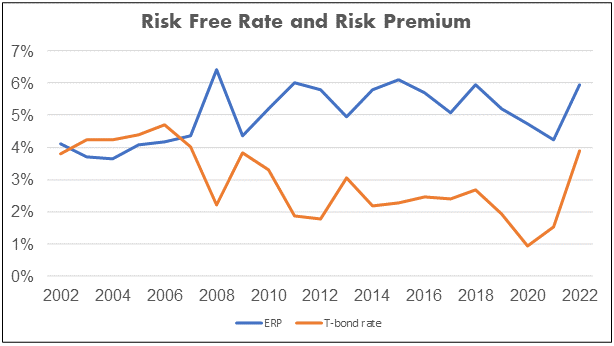Chart 4: US Risk-free rate and Equity Risk Premiums

Chart 5 illustrates the trends for the Beta of 4 key sectors in the US. You can see that both the Steel and Home Builders showed some cyclical patterns over the past 20 years.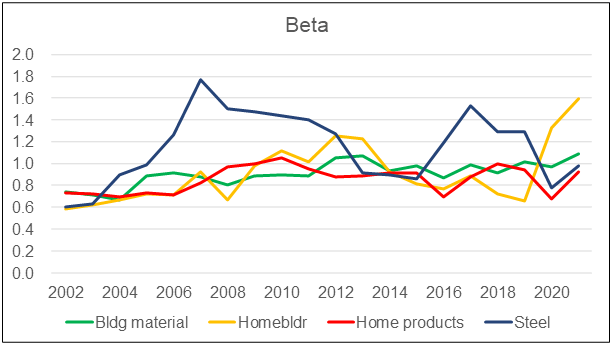Chart 5: Beta Trends for Selected Sectors in the US

All valuations are based on assumptions about the future. I will be the first to admit that in using the base rates, I am assuming that the historical values are good reflections of the future.

Looking at Charts 4 and 5, you can see that the metrics are volatile. It is better to use the normalized values than just the current values.

PS: The Charts are derived from the base rates data that I have compiled. If you need the actual numbers for your valuation purpose, email me. I will be most happy to share them with you.

## Debt

To determine the WACC, we need the DE ratio based on the market value of Debt.

We know that Debt can change over the cycle. I had to assume that the average DE over the cycle represents the normalized DE ratio.

There is another way to estimate the normalized Debt and DE ratio as follows.

In my valuation model, I have determined the normalized TCE based on the TCE/revenue ratio.

Given the normalized TCE we can then estimate the normalized Debt. This assumes that the TCE = SHF + Debt.

To determine the market value of Debt as per the bond model, I needed the Debt payment schedule. This can be obtained for the current situation. I can then determine the ratio between the Book Value of Debt and the Debt market value.

I next assumed that this ratio applies to the normalized Debt to derive the normalized market value of Debt.

## Impact of using cyclical values

In this section, I compared some of the discount rates used in my Seeking Alpha articles with those estimated using the normalized data. These will illustrate why having the base rates are important.

### Builders FirstSource

This is based on my Seeking Alpha article, “Builders FirstSource: Don't Be Mesmerized By The Past Few Years' Performance”. The WACC and the Cost of Equity were based on a Google Search. Refer to Table 1.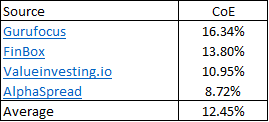Table 1: Builders FirstSource Cost of Equity

Cost of Equity = RFR + [(Unlevered Beta X Leverage) X ERP].

If I reverse-engineer the 12.45 %, I would have the following:

12.45 = 3.88 + [(1.09 X 1.33) X 5.9].

Where:

3.88 = 2022 Risk-free rate as per Damodaran.

1.09 = 2022 Unlevered Beta for the Building Materials sector as per Damodaran.

5.9 = 2022 Equity risk premium as per Damodaran.

1.33 = reverse-engineered leverage.

Now the cyclical factors based on 2006 to 2022 values are:

RFR = 2.46 %.

Unlevered Beta = 0.95.

ERP = 5.3 %.

Cost of Equity = 2.46 + [(0.95 X 1.33) X 5.3].

= 9.16 %.

You can see that the cyclical Cost of Equity is about 26 % lower than that based on the current values. This would provide an additional margin of safety based on the intrinsic value derived using the current values.

### US Steel

In my Seeking Alpha article, “U.S. Steel: A Different Group Than 9 Years Ago”, I assumed the cycle to be 2013 to 2021. I derived the WACC based on the CAPM using Damodaran 2021 data sets.

One of the scenarios I looked at was based on the data summarized in the center column of Table 2. (PS: I did not use this scenario in the Seeking Alpha article)

I obtained a WACC of 5.6 % based on the current discount rate. If I used the cyclical data, as summarized in the right column, I obtained a WACC of 7.7 %. Note that for this example, I changed the risk-free rates, Equity risk premium, and unleveled Beta. The cost of Debt and the D/E ratio remained unchanged.

In this case, the cyclical WACC was higher than the current one. This was because the cyclical Risk-free rate, Equity Risk Premium, and Beta were higher than the current ones.

A higher discount rate would mean a lower intrinsic value. The cyclical discount rate is 38 % higher than the current discount rate. Given that my normal margin of safety is 30%, using the cyclical discount rate will mean that there is no margin of safety.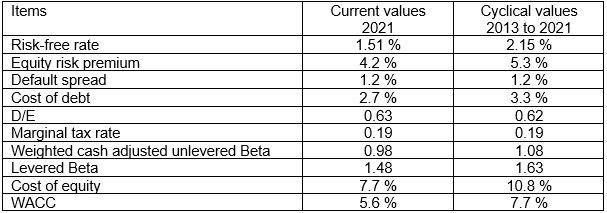Table 2: US Steel - Estimating the WACC

The results for US Steel are different from those of Builders FirstSource because of timing. The Builders FirstSource's data were from when the Ukraine invasion and high inflation were big concerns.

But the examples show that the difference between the cyclical discount rate and the current one is significant.

### NVR

This is a US Homebuilder covered in my Seeking Alpha article “NVR: A Good Jockey On A Strong Horse Riding Into A High Inflation Environment”. In this case, I took the cycle from 2005 to 2021.

The values used to determine the discount rate are shown in the center column of Table 3. In this example, I used the normalized DE ratio of 0.35 based on the average 2005 to 2021 values. This meant that the interest coverage ratio would change resulting in a higher Default spread. The result is a higher Cost of Debt.

But because the cyclical unlevered Beta is much lower than the current unlevered Beta, the WACC was reduced to 6.9 %. The cyclical WACC was lower than the one used by 15 %.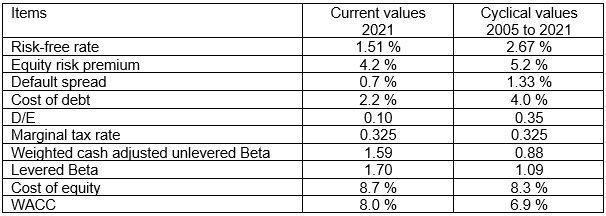Table 3: NVR - Estimating the WACC

The key challenge for NVR is determining the cyclical market cost of Debt. In the example shown, I used the cyclical DE ratio based on the Book Values. The CAPM uses the market cost of Debt / Market Equity value.  You can imagine the challenges in determining the cyclical DE ratio based on market values.

However, the impact of changes in the DE ratio using the Book Values is not that great as can be seen in Table 4. Here I compared the WACC with and without changes to the DE ratio. The changes here were based on the Book Values.

The results seem to suggest that we may not have to worry about the changes in the DE ratio.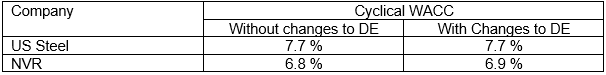Table 4: Comparative WACC with and without changes to the DE ratio.

## Conclusion

All valuations are based on assumptions. When valuing cyclical companies, we determine the normalized values to represent the future.

It is comparatively easy to derive the normalized earnings and cash flows. This is because the historical data are available from the various Annual Reports.

However, while the current WACC is readily available online, I could not find the historical WACC. This meant that I have to derive them. That is where the base rates come in.

I have also shown that there are significant differences between the current WACC and the normalized ones. To have a more accurate picture of the value of cyclical companies you should use the normalized WACC.

But as the examples have shown, even with the base rates there are DE assumptions required. As such it is more appropriate to use the cyclical discount rates qualitatively. This meant using it to indicate the direction of the change in the intrinsic value rather than compute the cyclical intrinsic values.